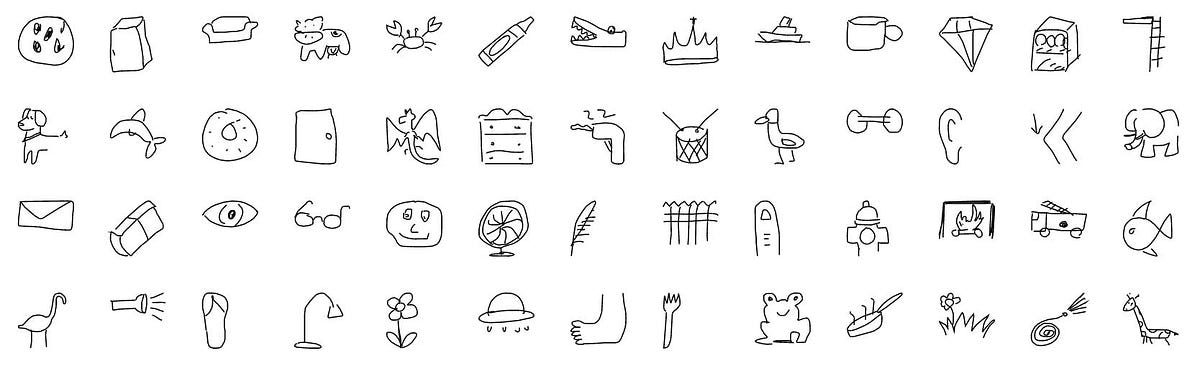# Introduction to CNNs Without using MNIST!Introduction to CNNs Without using MNIST! An introduction to CNN with an easily accessible dataset for beginners in deep learning.

## Introduction 🍵

The MNIST dataset is the most overused dataset for getting started with image classification. MNIST dataset comprising of 10-class handwritten digits introduced by Yann LeCun in 1998 come up over and over again, in scientific papers, blog posts, and so on. It contains 28×28 (also 32x32) grayscale images of handwritten digits, each with integers between 0 and 9.

The reason MNIST is so popular has to do with its size, allowing deep learning practitioners to quickly check, train, and publish their algorithms. There are certain variations and limitations of MNIST.

The main objective is to come up with a fresh dataset to understand Image Classification using CNNs.

## If not MNIST then what? 🤔

For the purpose of simple explanation, I have chosen Quick-Draw images by Google. These images are doodles that were generated in an A.I. experiment.

## PyTorch For Deep Learning — Convolutional Neural Networks ( Fashion-MNIST )

PyTorch For Deep Learning — Convolutional Neural Networks ( Fashion-MNIST ). This blog post is all about how to create a model to predict fashion mnist images and shows how to implement convolutional layers in the network.

## Satellite image classification with a convolutional neural network.

My latest project at Flatiron was to use neural networks to classify satellite image tiles. I chose to use a convolutional neural network (CNN).

## Image classification with PyTorch

CIFAR 10 Data set using logistic regression. In my previous posts we have gone through. Let us try to solve image classification of CIFAR-10 data set with Logistic regression.

## PyTorch for Deep Learning | Data Science | Machine Learning | Python

PyTorch for Deep Learning | Data Science | Machine Learning | Python. PyTorch is a library in Python which provides tools to build deep learning models. What python does for programming PyTorch does for deep learning. Python is a very flexible language for programming and just like python, the PyTorch library provides flexible tools for deep learning.

## Deep Learning and Neural Networks with Python and Pytorch

Hello and welcome to a Deep Learning and Neural Networks with Python and Pytorch tutorial starting from the basics. Building our Neural Network. Training Model. Convnet Intro. Training Convnet. On the GPU. Model Analysis